﻿ 通道等离子体环境对稳态等离子体推进器壁面腐蚀的影响 Effect of Tunnel Plasma Environment on Wall Erosion of Stationary Plasma Thruster

Mechanical Engineering and Technology
Vol.06 No.05(2017), Article ID:22999,11 pages
10.12677/MET.2017.65040

Effect of Tunnel Plasma Environment on Wall Erosion of Stationary Plasma Thruster

Huijun Cao1, Zhihao Zhang2

1School of Mechanical and Automation Engineering, Xiamen City University, Xiamen Fujian

2College of Materials, Xiamen University, Xiamen Fujian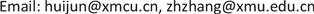Received: Nov. 18th, 2017; accepted: Dec. 5th, 2017; published: Dec. 13th, 2017ABSTRACT

The tunnel plasma environment can affect the distribution of high energy ion beam, and then the sputter-induced wall erosion. In this paper, the effects of preionization on the physical characteristic distributions and the wall erosion are investigated. The results indicate that the preionization do not affect the main physical characteristic in the discharge channel. While the wall erosion will be aggravated by the extra sputter plasma which is introduced by the preionization process. The effects of charge-exchange collision on the physical characteristic distributions and the wall erosion are also investigated. The results show that the ion density decreases and the wall erosion alleviates after taking the charge-exchange collision into consideration.

Keywords:Wall Erosion, Preionization, Charge-Exchange Collision, Stationary Plasma Thruster

1厦门城市职业学院，机械与自动化工程系，福建 厦门

2厦门大学，材料学院，福建 厦门1. 引言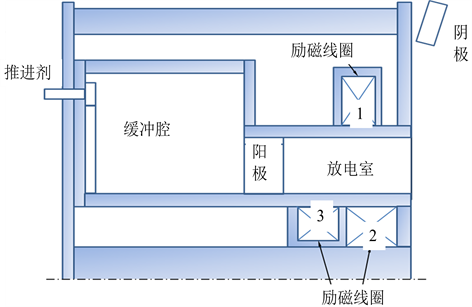Figure 1. The schematic of an aton-type stationary plasma thruster

Morozov等人通过实验手段测量了缓冲腔内氙离子光谱和腔内的电势，数据表明在缓冲腔内一定数量的氙原子被电离为氙离子，并且在腔内有大约1V的电势降  。于达仁等人的研究则表明缓冲区内的预电离现象能减低低频振荡幅值，并且随着预电离率的增加，低频振荡幅值降低   。以上对预电离的研究焦点都集中在预电离对低频振荡的影响方面，虽然预电离作用被证实能有效抑制低频振荡，使推进器获得更稳定的工作性能，但是缓冲腔及预电离的引入对推进器其他方面的影响，特别是壁面溅射腐蚀的影响至今还鲜有研究。

2. 仿真算法及模型设置

2.1. 全粒子PIC算法

$\nabla \cdot E=-\nabla \cdot \epsilon \nabla \Phi ={q}_{e}\left({n}_{i}-{n}_{e}\right)$ (1)

$F=m\frac{\text{d}v}{\text{d}t}=q\left(E+v×B\right)$ (2)

$\frac{\text{d}x}{\text{d}t}=v$ (3)

2.2. SPT通道仿真模型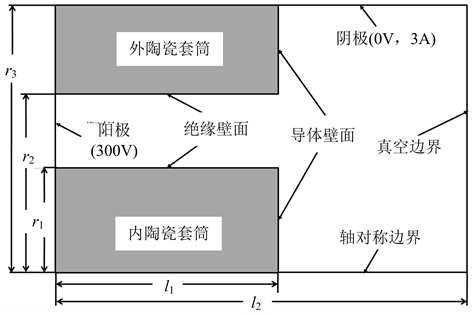Figure 2. Computational region and boundaries conditions used in the modelTable 1. Parameters of P70 Stationary Plasma Thruster

2.3. 预电离模型

${\beta }_{pre}=\frac{{n}_{i}{v}_{i}}{{n}_{i}{v}_{i}+{n}_{a}{v}_{a}}×100%$ (4)

2.4. 蒙特卡洛碰撞

${\omega }_{itot}={v}_{i}{Q}_{tot}{n}_{back}=\sum _{j=1}^{{N}_{c}}{\omega }_{j}=\sum _{j=1}^{{N}_{c}}{v}_{i}{Q}_{j}{n}_{back}$ (5)

${P}_{j}=1-\mathrm{exp}\left(-{\omega }_{\mathrm{max}}dt\right)\text{,}j=1,2,\cdots ,{N}_{c}$ (6)

${P}_{\mathrm{max}}=1-\mathrm{exp}\left(-{\omega }_{\mathrm{max}}dt\right)=1-\mathrm{exp}\left(-{v}_{\mathrm{max}}{n}_{a\mathrm{max}}{Q}_{\mathrm{max}}dt\right)$ (7)

2.5. 电荷交换模型

${\omega }_{cex}={c}_{r}{Q}_{cex}{n}_{a}$ (8)

Rapp认为同种物质的离子与背景原子发生电荷交换的碰撞截面是离子速度的函数，并给出了相应的表达式  ：

${Q}_{cex}={\left({k}_{1}\mathrm{ln}{v}_{i}+{k}_{2}\right)}^{2}$ (9)

Roy给出了氙离子与氙原子电荷交换碰撞情况下上式对应的参数值， ${k}_{1}=-0.8821×{10}^{-10}{\text{m}}^{2}$ 以及 ${k}_{2}=15.1262×{10}^{-10}{\text{m}}^{2}$  。

2.6. 壁面腐蚀估算模型

$q=\frac{{J}_{i}Y\left({E}_{i},{\theta }_{i}\right)\mathrm{cos}{\theta }_{i}}{{N}_{sub}}$ (10)

Sigmund通过理论推导证明了入射离子能量 ${E}_{i}$ 和角度 ${\theta }_{i}$ 对溅射产额 $Y$ 的影响是相互独立的，即溅射产额满足  ：

$Y\left({E}_{i},{\theta }_{i}\right)=Y\left(0\right)Y\left({\theta }_{i}\right)$ (11)

$q=\frac{{J}_{i}Y\left(0\right)\mathrm{cos}{\theta }_{i}}{{N}_{sub}}Y\left({\theta }_{i}\right)$ (12)

Katardjiev等人给出了二维坐标下两个方向的特征速度分量  ：

$\begin{array}{l}{V}_{x}=\frac{\text{d}x}{\text{d}t}=\kappa \left(Y\left({\theta }_{i}\right)-\frac{\text{d}Y\left({\theta }_{i}\right)}{\text{d}{\theta }_{i}}\mathrm{sin}{\theta }_{i}\mathrm{cos}{\theta }_{i}\right)\\ {V}_{y}=\frac{\text{d}y}{\text{d}t}=\kappa \frac{\text{d}Y\left({\theta }_{i}\right)}{\text{d}{\theta }_{i}}{\mathrm{cos}}^{2}{\theta }_{i}\end{array}$ (13)

Carter认为在二维轴对称坐标下，轴向 $z$ 和径向 $r$ 的特征速度分量依然满足上式  。对于SPT推进器而言，其壁面的溅射腐蚀模型通常是氙离子溅射轰击氮化硼陶瓷表面，Cheng  给出了此种情况下的能量溅射产额系数公式：

$Y\left(0\right)=\frac{A{E}_{i}^{0.474}}{1+B{E}_{i}^{0.3}}{\left(1-\sqrt{\frac{{E}_{th}}{{E}_{i}}}\right)}^{2.5}$ (14)

$Y\left({\theta }_{i}\right)={x}^{f}\mathrm{exp}\left[-\Sigma \left(x-1\right)\right]$ (15)

3. 仿真结果及讨论

3.1. 预电离对SPT推进器壁面腐蚀的影响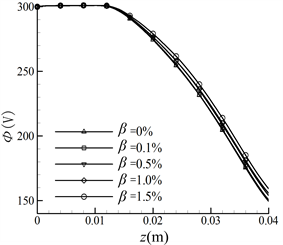(a)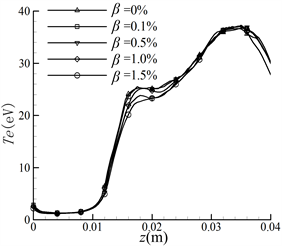(b)

Figure 3. Axial distribution of the physical properties with different pre-ionization ratios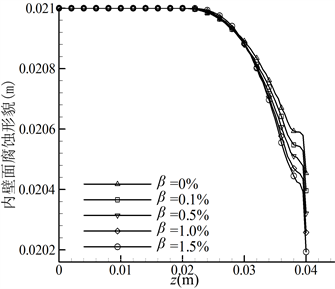Figure 4. The erosion profiles of channel walls with different pre-ionization ratios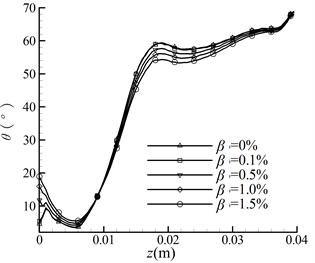(a)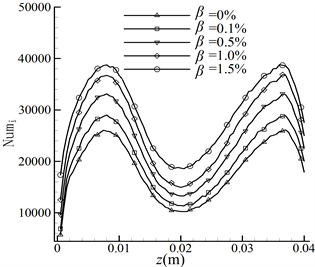(b)

Figure 5. Incidence parameters along the inner wall with different pre-ionization ratios

3.2. CEX碰撞对SPT推进器壁面腐蚀的影响

4. 结论Figure 6. Distribution of the ratio of CEX ions to beam ions $\left({n}_{cex}/{n}_{i}\right)$(a)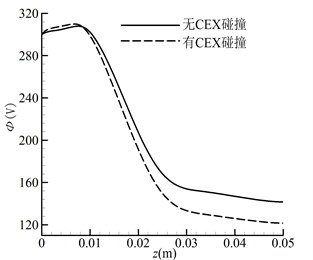(b)

Figure 7. Comparison of results with and without CEX collision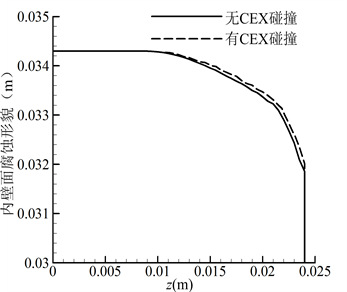Figure 8. Comparison of inner wall erosion profiles with and without CEX collision

Effect of Tunnel Plasma Environment on Wall Erosion of Stationary Plasma Thruster[J]. 机械工程与技术, 2017, 06(05): 337-347. http://dx.doi.org/10.12677/MET.2017.65040

1. 1. Cao, H., Li, Q., Shan, K., et al. (2015) Effect of Preionization on the Erosion of the Discharge Channel Wall in a Hall Thruster Using a Kinetic Simulation. IEEE Transactions on Plasma Science, 43, 130-140. https://doi.org/10.1109/TPS.2014.2367524

2. 2. 扈延林, 毛威, 李栋, 等. 面向全电推进卫星的霍尔推进技术[J]. 空间控制技术与应用, 2017, 43(1): 73-78.

3. 3. Goebel, D.M. and Katz, I. (2008) Fundamentals of Electric Propulsion: Ion and Hall Thrusters. John Wiley & Sons Inc. Publication, New Jersey, 325-443. https://doi.org/10.1002/9780470436448

4. 4. 钱中, 康小录, 王平阳, 等. 霍尔推力器等离子体羽流粒子模拟[J]. 上海航天, 2009, 26(4): 43-46.

5. 5. 康小录, 杭观荣, 朱智春. 霍尔电推进技术的发展与应用[J]. 火箭推进, 2017, 43(1):8-17.

6. 6. 吴汉基, 蒋远大, 张志远. 电推进技术的应用与发展趋势[J]. 推进技术, 2003, 24(5): 385-392.

7. 7. 毛根旺, 唐金兰. 航天器推进系统及其应用[M]. 西安: 西北工业大学出版社, 2009: 262-291.

8. 8. 李世忠, 陈虹. 现代小卫星技术与数字地球[J]. 遥感信息, 2000(4): 47-49.

9. 9. Martinez-Sanchez, M. and Pollard, J.E. (1998) Spacecraft Electric Propulsion—An Overview. Journal of Propulsion and Power, 14, 688-699. https://doi.org/10.2514/2.5331

10. 10. Fife, J.M., Martinez-Sanchez, M. and Szabo, J. (1996) A Numerical Study of Low-frequency Discharge Oscillations in Hall Thruster. 33th AIAA/SAE/ASME/ASEE Joint Propulsion Conference and Exhibit, AIAA-1997-3052, Seattle, Washington.

11. 11. Furukawa, T., Miyasaka, T. and Fujiwara, T. (2005) Control of Low-Frequency Oscillation in a Hall Thruster. Transactions of the Japan Society for Aeronautical and Space Sciences, 44, 164-170. https://doi.org/10.2322/tjsass.44.164

12. 12. Tamida, T., Nakagawa, T., Suga, I., et al. (2007) Determining Parameter Sets for Low-Frequency-Oscillation-Free Operation of Hall Thruster. Journal of Applied Physics, 102, 043304-1-043304-6. https://doi.org/10.1063/1.2771039

13. 13. Barral, S. and Ahedo Galilea, E. (2009) Low-Frequency Model of Breathing Oscillations in Hall Discharges. Physical Review E, 79, 046401-1-046401-11. https://doi.org/10.1103/PhysRevE.79.046401

14. 14. Yu, D.R., Wei, L.Q., Zhao, Z.Y., et al. (2008) Effect of Preionization in Aton-Type Hall Thruster on Low Frequency Oscillation. Physics of Plasmas, 15, 043502-1-043502-6.

15. 15. 韩轲, 魏立秋, 纪延超. P70霍尔推力器新型缓冲腔磁路对预电离及放电的影响[J]. 推进技术, 2011, 32(6): 823-827.

16. 16. Morozov, A.I., Bugrova, A.I. and Desyatskov, A.V. (1997) ATON-Thruster Plasma Accelerator. Plasma Physics Reports, 23, 587-597.

17. 17. Yu, D.R., Wang, C.S., Wei, L.Q., et al. (2008) Stabilizing of Low Frequency Oscillation in Hall Thrusters. Physics of Plasmas, 15, Article ID: 113503.

18. 18. Szabo, J.J. (2001) Fully Kinetic Numerical Modeling of a Plasma Thruster. Thesis for the Degree of Doctor of Philosophy, Massachusetts Institute of Technology, Cambridge, 79-126.

19. 19. Parra, F.I., Ahedo, E., Fite, J.M., et al. (2006) A Two-Dimensional Hybrid Model of the Hall Thruster Discharge. Journal of Applied Physics, 100, Article ID: 023304. https://doi.org/10.1063/1.2219165

20. 20. Sommier, E., Scharfe, M.K., Gascon, N., et al. (2007) Simulating Plasma-Induced Hall Thruster Wall Erosion with a Two-Dimensional Hybrid Model. IEEE Transactions on Plasma Science, 35, 1379-1387. https://doi.org/10.1109/TPS.2007.905943

21. 21. 刘辉, 吴勃英, 鄂鹏, 等. ATON型Hall推力器缓冲区预电离问题研究[J]. 物理学报, 2010, 59(10): 7203-7207.

22. 22. Vahedi, V. and Surendra, M. (1995) A Monte Carlo Collision Model for the Particle-in-Cell Method: Applications to Argon and Oxygen Discharges. Computer Physics Communications, 87, 179-198. https://doi.org/10.1016/0010-4655(94)00171-W

23. 23. Kafafy, R.I. (2005) Immersed Finite Element Particle-in-Cell Simulations of Ion Propulsion. Thesis for the Degree of Doctor of Philosophy, University of Virginia Polytechnic Institute and State, Virginia, 29-55.

24. 24. Rapp, D. and Francis, W.E. (1962) Charge Exchange between Gaseous Ions and Atoms. Journal of Chemical Physics, 37, 2631-2645. https://doi.org/10.1063/1.1733066

25. 25. Roy, R.I.S., Hastings, D.E. and Gatsonis, N.A. (1996) Ion-Thruster Plume Modeling for Backﬂow Contamination. Journal of Spacecraft and Rockets, 33, 525-534. https://doi.org/10.2514/3.26795

26. 26. Sigmund, P. (1969) Theory of Sputtering. I. Sputtering Yield of Amorphous and Polycrystalline Targets. Physical Review, 184, 383-416. https://doi.org/10.1103/PhysRev.184.383

27. 27. Katardjiev, I.V., Carter, G. and Nobes, M.J. (1988) Precision Modeling of the Ask-Substrate Evolution during Ion Etching. Journal of Vacuum Science and Technology, A6, 2443-2450. https://doi.org/10.1116/1.575570

28. 28. Carter, G. (1997) Huygen’s Wavelets and Deterministic Evolution of Surfaces and Interfaces. Vacuum, 48, 925-931. https://doi.org/10.1016/S0042-207X(97)00089-4

29. 29. Cheng, S.Y.M. (2007) Modeling of Hall Thruster Lifetime and Erosion Mechanisms. Thesis for the Degree of Doctor of Philosophy, Massachusetts Institute of Technology, Cambridge, 39-53.

30. 30. Yamamura, Y. (1984) An Empirial Formula for Angular Dependence of Sputtering Yields. Radiation Effects, 80, 57-72. https://doi.org/10.1080/00337578408222489

31. 31. Yokota, S., Sakoh, D., Matsui, M., et al. (2008) Charge Exchange Ion Number Density Distribution in Hall Thruster Plume. Vacuum, 83, 57-60. https://doi.org/10.1016/j.vacuum.2008.03.024